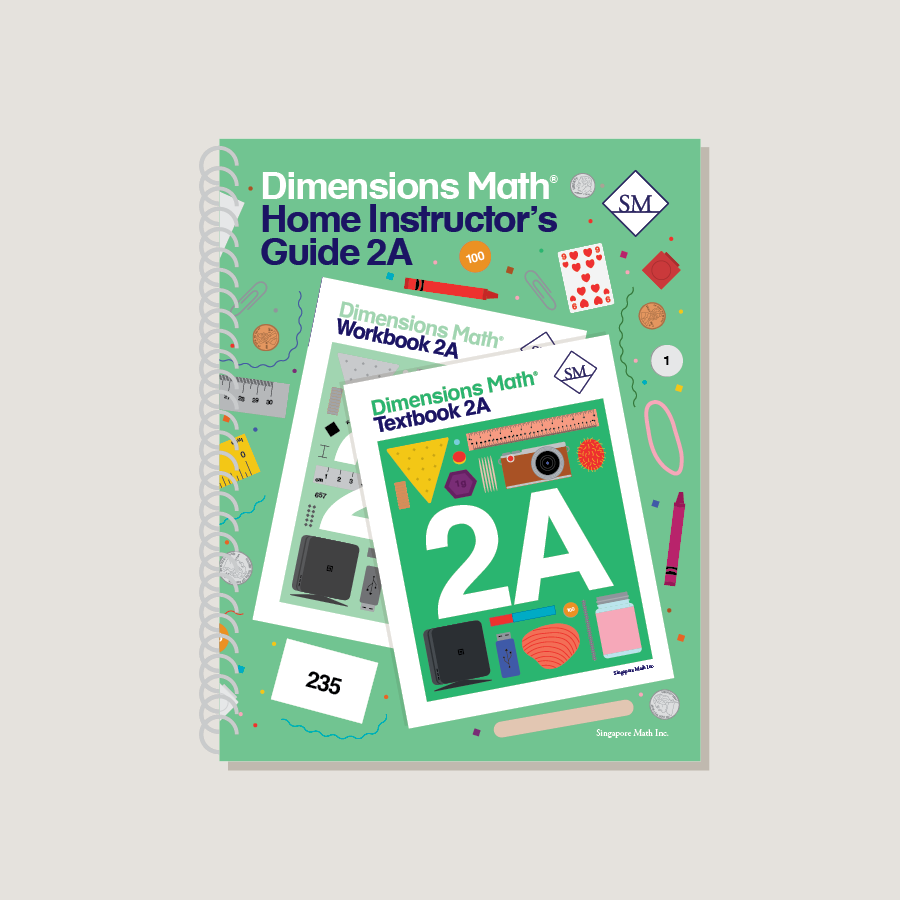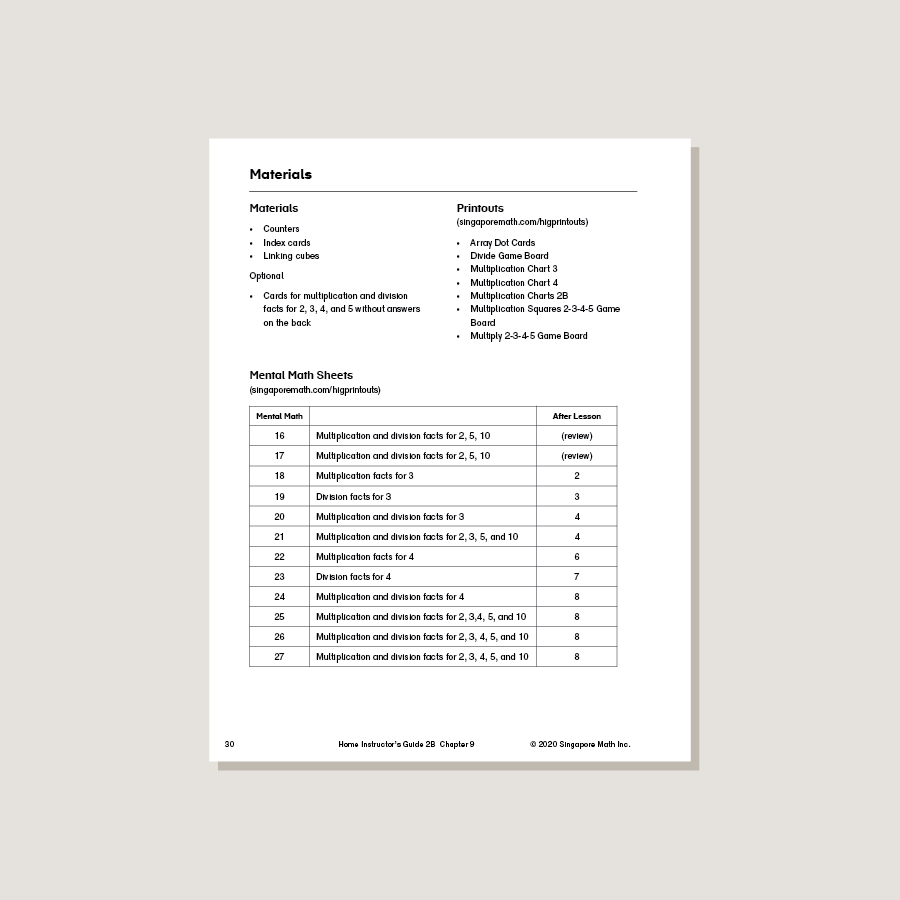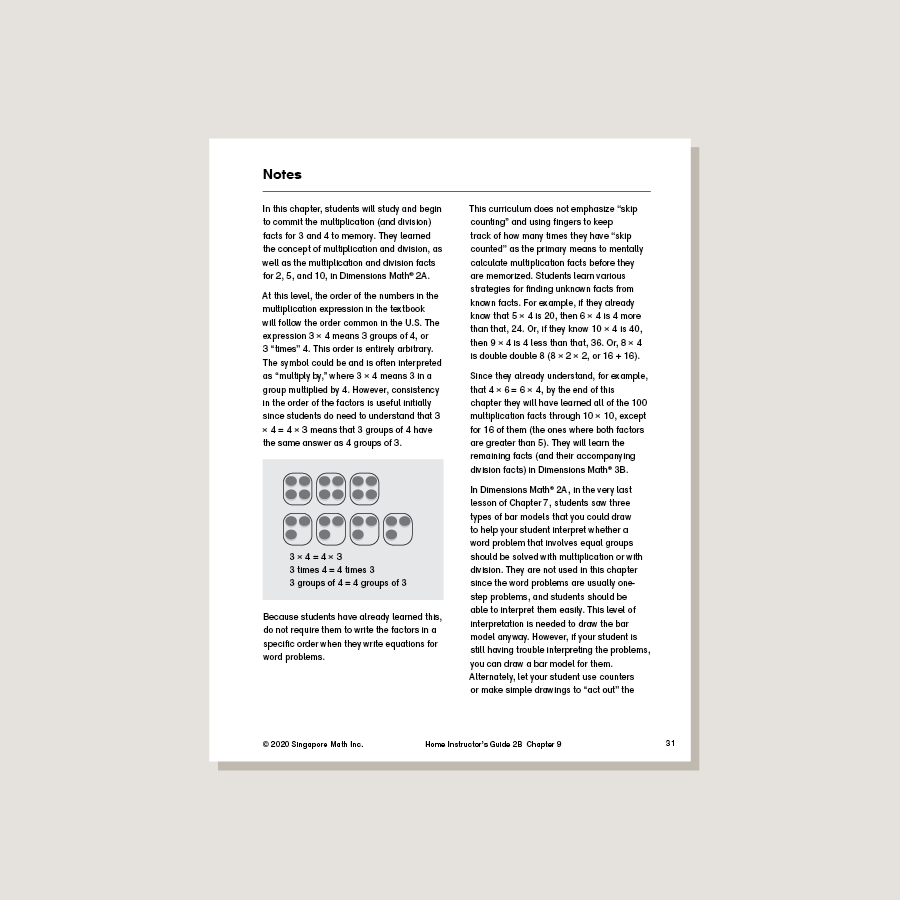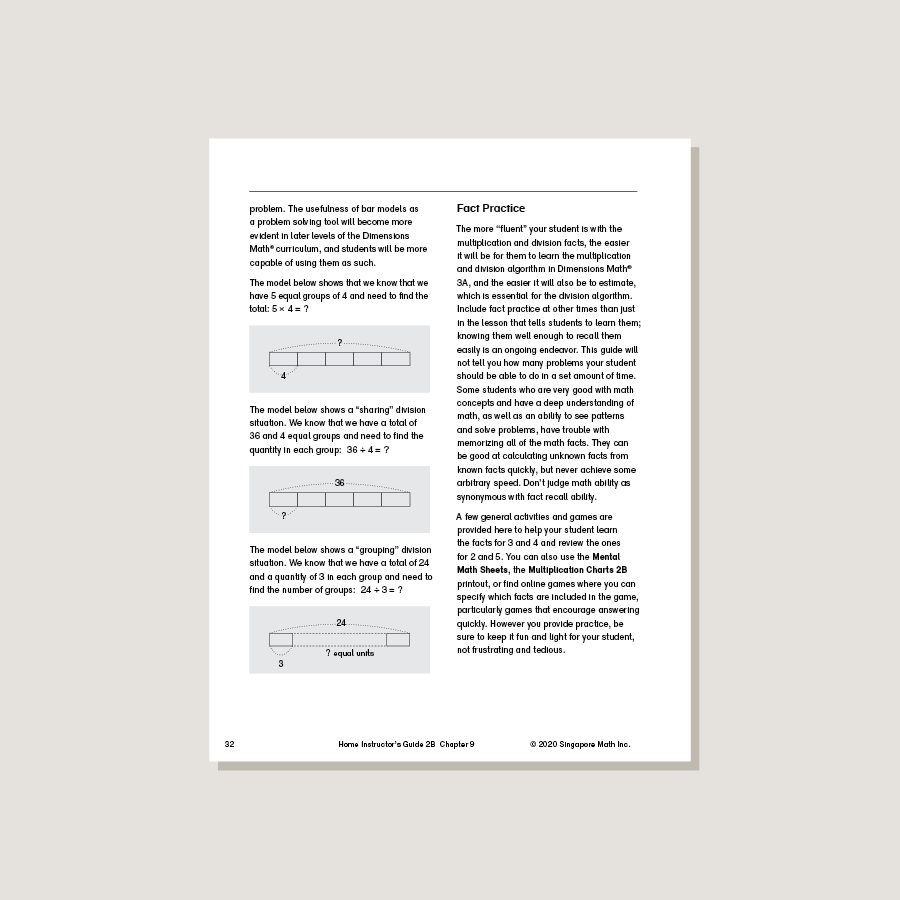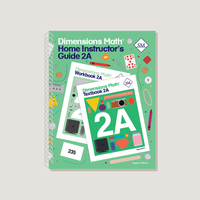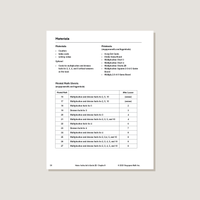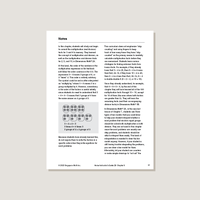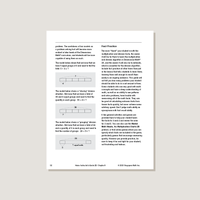# Dimensions Math Home Instructor's Guide 2A

\$21.40

Dimensions Math Home Instructor’s Guides are an essential resource for teaching Dimensions Math in a home or one-on-one setting. The purpose of the Guides is to help educators understand concepts in the Dimensions Math curriculum and teach as effectively as possible. They include extensive background notes, lesson suggestions, tips, and activities. Home Instructor’s Guides include answer keys for corresponding Dimensions Math Textbooks and Workbooks.

Note: Soft cover, spiral bound.

SKU: DMHIG2A

ISBN: 9781947226609

Pagecount: 154

Dimensions: 8.5 x 11 x 0.75 in

Binding: Spiral

Color: Grayscale

Cover: Soft

Perforated: No

Sample Pages

Chapter 1: Numbers to 1,000
Lesson 1: Tens and Ones
Lesson 2: Counting by Tens or Ones
Lesson 3: Comparing Tens and Ones
Lesson 4: Hundreds, Tens, and Ones
Lesson 5: Place Value
Lesson 6: Comparing Hundreds, Tens, and Ones
Lesson 7: Counting by Hundreds, Tens, or Ones
Lesson 8: Practice

Chapter 2: Addition and Subtraction — Part 1
Lesson 2: Strategies for Subtraction
Lesson 3: Parts and Whole
Lesson 4: Comparison
Lesson 5: Practice

Chapter 3: Addition and Subtraction — Part 2
Lesson 2: Subtraction Without Regrouping
Lesson 3: Addition with Regrouping Ones
Lesson 4: Addition with Regrouping Tens
Lesson 5: Addition with Regrouping Tens and Ones
Lesson 6: Practice A
Lesson 7: Subtraction with Regrouping from Tens
Lesson 8: Subtraction with Regrouping from Hundreds
Lesson 9: Subtraction with Regrouping from Two Places
Lesson 10: Subtraction with Regrouping across Zeros
Lesson 11: Practice B
Lesson 12: Practice C

Chapter 4: Length
Lesson 1: Centimeters
Lesson 2: Estimating Length in Centimeters
Lesson 3: Meters
Lesson 4: Estimating Length in Meters
Lesson 5: Inches
Lesson 6: Using Rulers
Lesson 7: Feet
Lesson 8: Practice

Chapter 5: Weight
Lesson 1: Grams
Lesson 2: Kilograms
Lesson 3: Pounds
Lesson 4: Practice
Review 1

Chapter 6: Multiplication and Division
Lesson 1: Multiplication — Part 1
Lesson 2: Multiplication — Part 2
Lesson 3: Practice A
Lesson 4: Division — Part 1
Lesson 5: Division — Part 2
Lesson 6: Multiplication and Division
Lesson 7: Practice B

Chapter 7: Multiplication and Division of 2, 5, and 10
Lesson 1: The Multiplication Table of 5
Lesson 2: Multiplication Facts of 5
Lesson 3: Practice A
Lesson 4: The Multiplication Table of 2
Lesson 5: Multiplication Facts of 2
Lesson 6: Practice B
Lesson 7: The Multiplication Table of 10
Lesson 8: Dividing by 2
Lesson 9: Dividing by 5 and 10
Lesson 10: Practice C
Lesson 11: Word Problems
Review 2

A & B Books: Our programs divide the school year into two semesters. “A” level books are for the first half of the school year. “B” level books are for the second half of the school year. You need both “A” and “B” material for a complete school year.

Required Components: Textbooks, Workbooks, and Guides (either Home Instructor’s Guides or Teacher’s Guides) are all necessary components. These three elements each serve a unique function and work together to build math mastery.

Printouts: Dimensions Math PK-5 Resources

Recommended Manipulatives: Dimensions Math Grades PK-5 Recommended Manipulatives